Gradient-augmented hybrid interface capturing method for incompressible two-phase flow
Institution of Applied Physics and Computational Mathematics, Beijing 100094, China
LTCS and Department of Mechanics & Aerospace Engineering, College of Engineering, Peking University, Beijing 100871, China
Center for Applied Physics and Technology, Peking University, Beijing 100871, China

† Corresponding author. E-mail: kliu@pku.edu.cn

Project supported by the National Natural Science Foundation of China (Grant Nos. 10972010, 11028206, 11371069, 11372052, 11402029, and 11472060), the Science and Technology Development Foundation of China Academy of Engineering Physics (CAEP), China (Grant No. 2014B0201030), and the Defense Industrial Technology Development Program of China (Grant No. B1520132012).

Abstract
Abstract

Motivated by inconveniences of present hybrid methods, a gradient-augmented hybrid interface capturing method (GAHM) is presented for incompressible two-phase flow. A front tracking method (FTM) is used as the skeleton of the GAHM for low mass loss and resources. Smooth eulerian level set values are calculated from the FTM interface, and are used for a local interface reconstruction. The reconstruction avoids marker particle redistribution and enables an automatic treatment of interfacial topology change. The cubic Hermit interpolation is employed in all steps of the GAHM to capture subgrid structures within a single spacial cell. The performance of the GAHM is carefully evaluated in a benchmark test. Results show significant improvements of mass loss, clear subgrid structures, highly accurate derivatives (normals and curvatures) and low cost. The GAHM is further coupled with an incompressible multiphase flow solver, Super CE/SE, for more complex and practical applications. The updated solver is evaluated through comparison with an early droplet research.

PACS: 47.11.–j;47.55.D–
1. Introduction

Accurate and efficient simulation of interface evolution plays an important role for a wide range of computational fluid dynamics (CFD) problems, especially for multiphase flow. It is even more crucial for problems involving derivatives such as normals or curvatures. For example, the accurate calculation of the interfacial tension, which is highly sensitive to curvatures, is essential for the simulation of droplets or bubbles. Various numerical methods have been proposed to describe the evolution of the interface. These methods are further divided into eulerian capturing methods (ECMs) (e.g. the level set method (LSM)), which implicitly capture the interface, and lagrangian tracking methods (LTMs) (e.g. the front tracking method (FTM)), which explicitly track the interface.

ECMs capture smooth interface with high accuracy and automatically handle interface topology change such as merging or breaking. Nevertheless, ECMs suffer inconveniences in the following aspects: losses of subgrid structures, inaccurate approximations of normals and curvatures, and large computational stencils required by high order numerical methods. The LTM provides sharp interface with high mass conservation. However, the application to three-dimensional (3D) problems is difficult. Main inconveniences include the inefficient interface reconstruction and complicated calculation of interface topology change.

In order to combine advantages of different methods, popular and effective hybrid methods, such as the hybrid particles level set method (HPLSM), have been proposed. However, they are based on ECMs and inherit common inconveniences of ECMs. Moreover, key procedures of these schemes include dissipative interpolation on fixed grids. This kind of interpolation may smooth out important sharp details.

Another possible strategy for improving interface resolution is to employ gradient information. Gradient-augmented methods (GAM), including the compact scheme and the space time conservation element and solution element (CE/SE) method, show that gradients can significantly improve efficiency, accuracy and compactness. The compactness also provides a simple treatment of adaptive mesh refinement and boundary conditions. GAMs achieve success in a wide range of computational fluid dynamics problems. As far as we know, few papers[13,14] report applications of this strategy for capturing interface.

Motivated by inconveniences of hybrid methods, a gradient-augmented hybrid method (GAHM) is presented for capturing interfaces in an incompressible two-phase flow. The method uses a modified FTM as its skeleton and combines the LSM and cubic Hermit interpolation (CHI). Gradients are coupled in all steps of the GAHM to maintain high resolution within a single spatial cell. Smooth eulerian level set values are calculated from the interface. They are used for physical simulation as done in the LSM and also for a local eulerian interface reconstruction. The reconstruction avoids marker particles redistribution and enables an automatic treatment of interfacial topology change.

In the next section, we will briefly present the numerical approach used for simulating an incompressible two-phase flow. Then we describe the GAHM in Section 3. Finally, we demonstrate properties of the GAHM including accuracy, convergence and cost.

2. Incompressible two-phase flow approach

Consider two incompressible fluids, fluid 1 with density ρ1 and viscosity μ1 and fluid 2 with density ρ2 and viscosity μ2. Let σ denote the surface tension coefficient. The dimensionless governing equations for two-phase incompressible flow with interfacial forces are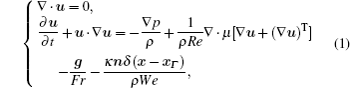where u, ρ, p, μ, g, n, and κ denote the velocity, density, pressure, viscosity, gravity, outward unit normal vector, and curvature, respectively; δ(xxΓ) is a delta function that is zero everywhere except at the interface where x = xΓ; Re = ρ1UL/μ1, Fr = U2/gL, and We = ρ1U2L/σ denote the Reynolds, Froude, and Weber numbers, respectively. To prevent numerical instability, discontinuous variables ρ and μ are smoothed as ρ = ρ2/ρ1 + (1 − ρ2/ρ1)H(φ) and μ = μ2/μ1 + (1 − μ2/μ1) with H(φ) denoting a heaviside function of ϕ, which is defined by a level set function as follows: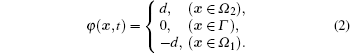In Eq. (2), Ω1 is the region bounded by the interface Γ and Ω2 is the region outside Ω1. The total computational domain is the union of Ω1, Ω2, and Γ. d denotes the shortest distance from point x to the interface Γ. φ is governed by the level set equation and captured with the HPLSM or GAHM. Normal vector n and curvature κ are derived as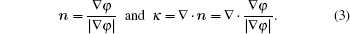By employing the continuum surface force (CSF) model, The interface force is reformulated as a volume force κ(φ)φδ(φ)/We with δ(φ) = dH(φ)/dφ and κ(φ) = (φ/|φ|). H(φ) is written as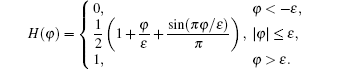Equation (1) is solved using an explicit variable-density projection method. The main idea is to first predict an intermediate velocity u* by splitting Eq. (1) into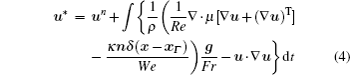and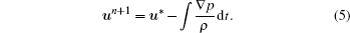The u* satisfies physical boundary condition. Assume ∇ · un + 1 = 0 and take divergence of Eq. (5), then we will have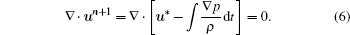Pressure can be evaluated form Eq. (6) and boundary condition n · p = 0. Then, pressures are submitted into Eq. (5), and finally velocities are obtained.

As shown in Fig. 1, simulations are performed on a rectangular eulerian domain with velocity at grid nodes and pressure at centriods of grid cells. Equation (4) is solved with an improved CE/SE scheme. Please refer to Ref.  for details. Equations (5) and (6) are calculated with the second order centered difference scheme and a second order Runge-Kutta method (RKM).

 Figure Option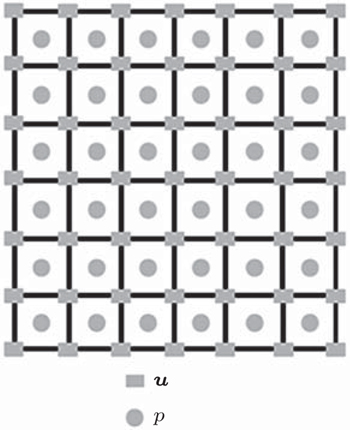Fig. 1. Distribution of flow variables.

In the GAHM, the lagrangian interface is composed of points and cubic curves. A curve is governed by a CHI equation and stored as a line segment. The two end points of the line segments are called markers. Unlike the FTM, the GAHM also evolves level set value φ and its gradient ψ on each marker. The FTM interface reconstruction is replaced with a local eulerian reconstruction by using the level set values. The eulerian reconstruction eliminates marker redistribution and enables an automatic treatment of interfacial topology change. During simulation, firstly, the interface is evolved with a characteristic method. Secondly, eulerian level set values are projected from the interface for physical calculations. Finally, the interface is locally reconstructed with eulerian level set values.

In the GAHM, the interface is evolved by solving the lagrangian level set equation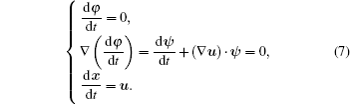Equation (7) describes the evolution of the level set function φ and its gradient ψ along the characteristic curve defined by dx/dt = u. Like the FTM, equation (7) is discretized with a characteristic method and the RKM. Velocities and their gradients are approximated with the CHI in eulerian grid cells. Readers can consult Ref.  on the CHI.

3.2. Calculation of eulerian level set values

Eulerian level set values are calculated from the tracked interface. Figure 2 shows the details of the calculation. As the interface deforms, interfacial curves become non-uniform. In some parts, markers are crowded. Line segment provides enough resolution. In other parts, markers are loose. Line segment is inadequate. The accurate CHI equation is more appropriate. Refer to Fig. 2. Firstly, curves with length above 1.5 times eulerian spatial step h are identified such as curve PQ in Fig. 2, and then PQ is divided into three line segments PP0, P0P1, and P1Q. Points P0 and P1 are yielded using the CHI in line segment PQ. Q0 and Q1 are points of tri-section of line segment PQ. Then, level set values are calculated from line segments PP0, P0P1, and P1Q. Take line segment P0P1 for example. For a grid node M, we project vector P0M onto line segment P0P1, yielding vector P0PM and point PM. Local level set values near P0P1 is determined by

 Figure Option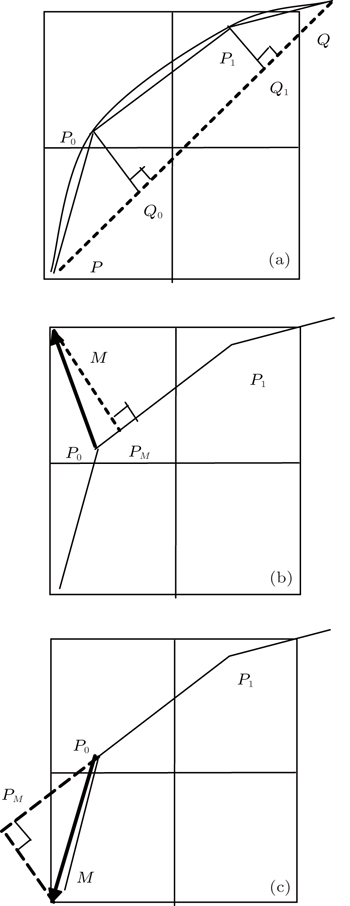Fig. 2. Calculations of eulerian level set values from curve PQ: (a) division of curve PQ, (b) PM in line segment P0P1, and (c) PM outside line segment P0P1.

if (PM is in line segment P0P1)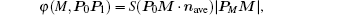else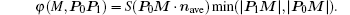nave is the average of normals at points P0 and P1. Fuction S(x) is defined as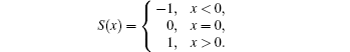According to the above code, an eulerian grid node has several level set values corresponding to different line segments. Refer to Fig. 3. Taking grid node M for example, we draw a circle of radius 1.5 h centered at M, here h is the eulerian spatial step. The circle covers five interfacial points (P1P5) and 6 line segments (P0P1P5P6). Six level set values are yielded. The final level set value is the one with minimum absolute value (φ(M) = φ(M,P2 P3)) Geometric information (such as n and κ) is approximated with eulerian level set values as done in the LSM. An obvious observation is that all derived level set values exactly follow the definition of the level set function. Moreover, level set values are independent of eulerian datum. Hence, the GAHM is capable of performing the adaptive simulations and calculations on unstructured grids.

 Figure Option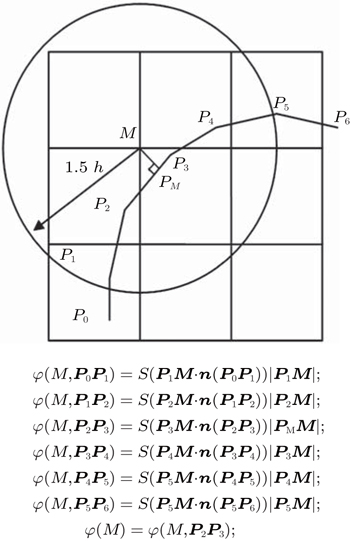Fig. 3. Computations of level set value on eulerian node M.
3.3. Local uulerian interface reconstruction

As the interface deforms, the distances of neighboring markers are non-uniform. This may cause extra resources, where markers are crowded, or loss of the subgrid structures, where markers are insufficient. Numerical errors may also lead to oscillations on the tracked interface. This problem is hard to handle by the FTM, particularly difficult in 3D applications. Du et al. presented three methods: grid free tracking (GF), grid-based tracking (GB), and locally grid-based tracking (LGB). The GF reconstructs an interface with information about markers. It is accurate, but expensive and inefficient. The GB reconstructs an interface with crossings of the interface with the fixed grid edges. It is robust but dissipative. The LGB combines GF with GB. It uses the GF for interface propagation, and the GB for local interface reconstruction in bifurcating regions. The concept of the LGB is borrowed and combined with the CHI. We call the modified LGB the gradient-augmented LGB (GALGB).

Figure 4 illustrates steps of the GALGB. In step 1, the bifurcating region is detected via the distance of the neighboring markers and the angle of neighboring line segments. Then all the markers in the bifurcating region are removed (makers M and N). In step 2, a new line segment is generated by connecting boundary markers of the bifurcating region (line segment PQ). Points of n-section on the new line segment are used as intermediate points for step 3 (points I0 and I1). The n is the number of crossing eulerian cells of the new line segment. The φ and ψ on intermediate points are calculated using the CHI in eulerian cells. In step 3, the position of new markers xnm and corresponding variables are determined by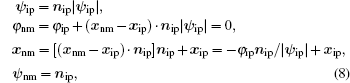where subscripts nm and ip denote variables on new marker and intermediate point, respectively. Finally all new markers are connected and then added to the interface. Since the tracked interface is reconstructed with eulerian datum, the interfacial topology changes are naturally and automatically handled.

 Figure Option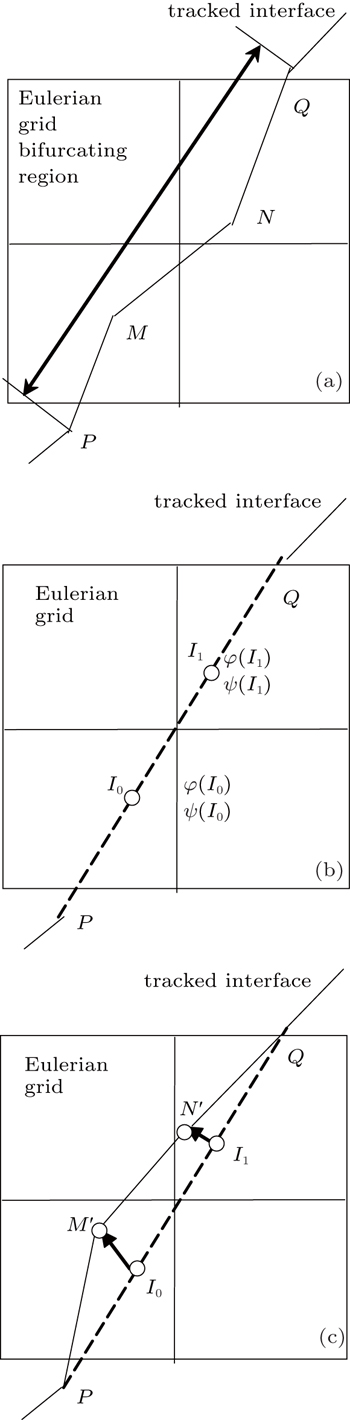Fig. 4. Steps of the gradient-augmented locally grid based tracking GALGB: (a) original interface, (b) intermediate points I0 and I1, (c) the reconstructed interface.
4. Numerical examples
4.1. Single vortex in a box

A difficult case is used to examine the capability of capturing subgrid structures. The initial interface is a circle of radius 0.15 centered at (0.5, 0.75) in a unit square with grid size 100 × 100. The prescribed velocity field is defined as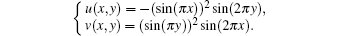This velocity field stretches the circle into a very thin, spiral filament, which is very difficult to capture on the fixed grids. For example, the area obtained by the LSM on a grid of size 64 × 64 completely disappears. In order to analyze the accuracy, the velocity field is reversed at t = 8 when the interface reaches a maximal deformation. Then the circle is recovered at t = 16. In this test, the reconstruction of the interface is an essential part of the scheme.

Figures 5(a)5(d) show the interfaces at t = 0, t = 4, t = 8, and t = 16, respectively. Thin spiral filament is well captured on a scale of the grid spacing by the GAHM, while serious mass losses are observed at both the head and tail of the filament for the HPLSM. According to Fig. 5(c), the HPLSM loses about two revolutions on the receding tail of the structure and recovers only 97.3% of the initial circle. While the GAHM only loses half revolution and recovers 99.98% of the whole circle. Also note that in Fig. 5(b) the HPLSM solution near (0.6, 0.2) breaks up into several components. The error is induced by inaccurately “reseeded” marker particles (we call them markers in short). In contrast, the GAHM recovers a connected structure at the same domain where the width of the interface is about a grid cell.

 Figure Option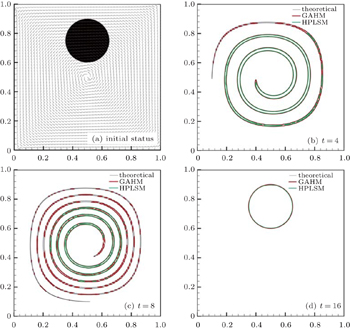Fig. 5. Evolutions of the interface on a 100 × 100 grid.

To further investigate grid dependence and accuracy, the values of considered grid cell spacing l are 1/50, 1/100, 1/200, and 1/400, respectively. Table 1 shows the lost area at t = 16 and particle numbers at t = 0 and 8 respectively. Errors by the GAHM are indicated to be close to second-order convergence of the lost area. Particle number reveals that the GAHM saves over 90% of memories, which are occupied by particles in the HPLSM. The growth rate of np (the number of marker particles at t = 8) with respect to l in the GAHM is smaller than that in the HPLSM. We can easily prove it by deriving the relationship between np and l. The reason is that marker particles in the GAHM describe a curve while particles in the HPLSM describe a local domain. This feature can help save memories and increase efficiency. Note that the lost area for HPLSM with grid cell spacing 1/50 is significantly large. It implies that either the grid resolution or distributed particles are insufficient.Table 1.

Relative errors of presverved area from different methods.

.

Table 2 shows errors of both level set function and its derivatives at t = 16. The GAHM guarantees approximately third order accurate level set function and second order accurate derivatives. While the HPLSM is only first order accurate for all information.Table 2.

Accuracies and convergences of different methods.

.
4.2. Single droplet falling

A symmetrical and laminar 2D droplet falling case is employed to evaluate the performance of the GAHM for incompressible multiphase flows. The computational domain is a rectangle with 100 × 200 uniform grid cells. The boundary condition is non-slip wall. The three numbers are Re = 100, Fr = 1, and We = 50. Density and viscosity ratios are 1.125 and 50. The interface is captured with the HPLSM or GAHM. An initial stationary circular droplet with a radius of 1 is centered at (4.5, 16.5) inside a fluid. Driven by the gravity, the droplet falls toward the bottom and induces several vortices. During the falling, it deforms and stretches into a dumbbell shape. The handle of the final shape at the time of 105 is much thinner than the size of a grid cell and it is very difficult to capture.

Table 3 shows the dependence on the initial number of markers. The GAHM is more efficient (approximately 20% ∼65% of the CPU time by the HPLSM) and accurate (conserving more area) than the HPLSM. It costs less memories (approximate 2.5% ∼8% of the particles in the HPLSM) too. All schemes in Table 3 conserve similar areas. Hence, 280 initial particles are sufficient for this case.Table 3.

Areas and particle numbers of the GAHM and HPLSM (Initial area is 3.14).

.

In Fig. 6, our numerical results are compared with original results. The droplet by our approach remains connected and symmetrical. The comparison implies that our approach is less dissipative and preserves more subgrid structures.

 Figure Option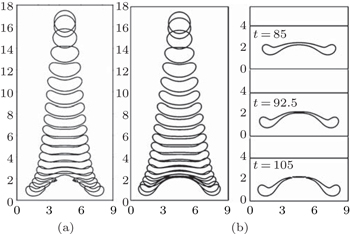Fig. 6. Droplets obtained from (a) Ref.  and (b) the GAHM.
5. Conclusions

Based on a combination of the accurate FTM, smooth LSM and compact CHI, a gradient-augmented interface capturing method is presented for an incompressible two-phase flow. Gradient is of great help to reduce numerical error and to optimize computational stencil. Smooth and sharp level set contours are projected from the tracked interface. As a result, normals are approximated more easily and accurately. The local eulerian interface reconstruction eliminates marker redistribution and automatically handles interfacial topology changes. Test results demonstrate significant improvements on mass loss, highly accurate derivatives, clear subgrid structures, and low cost.

The GAHM is further coupled with an incompressible two-phase flow, Super CE/SE, for more practical evaluations. The updated solver maintains a sharp dumbbell-shaped interface, which ensures correct interfacial tension and physical characteristics. Results reveal that the GAHM is capable of accurately simulating the incompressible two phase flow with complex interface deformation.

Reference
 1 Osher SSethian J A 1988 J. Comput. Phys. 79 12 2 Du JFix BGlim JJia X CLi X LLi Y HWu L L 2006 J. Comput. Phys. 213 613 3 Enright DFedkiw RFerziger JMitchell I 2002 J. Comput. Phys. 176 205 4 Adam Y 1977 J. Comput. Phys. 24 10 5 Chang S C 1995 J. Comput. Phys. 119 295 6 Wang GZhang D LLiu K X 2011 Comput. Phys. Commun. 182 1589 7 Shen HLiu K XZhang D L 2011 Chin. Phys. Lett. 28 124705 8 Chen Q YWang J TLiu K X 2010 J. Comput. Phys. 229 7503 9 Chen Q YLiu K X 2012 Comput. Fluids 56 92 10 Fu ZLiu K X 2012 Chin. Phys. 21 040202 11 Fu ZLiu K XLuo N 2014 Chin. Phys. 23 020202 12 Wang J T2007“High-order Scheme of Space-Time Conservation Element and Solution Element Method and Its Applications”Ph. D. ThesisBeijingPeking University(in Chinese) 13 Yabe TXiao F 1995 Comput. Math. Appl. 29 15 14 Nave J CRosales R RSeibold B 2010 J. Comput. Phys. 229 3802 15 Sussman MSmereka POsher S 1994 J. Comput. Phys. 114 334 16 Bell J BMarcus D L 1992 J. Comput. Phys. 101 146 17 Shu C W1997NASA/CR-97-20625310.1007/BFb0096355 18 Strain J 2000 J. Comput. Phys. 161 512 19 Bell JColella PGlaz H 1989 J. Comput. Phys. 85 257 20 Ni M JKomori SMorley N B 2006 Int. J. Heat Mass Transfer 49 366